Latest Banking jobs   »   Quantitative Aptitude Quiz For ESIC- UDC,...

# Quantitative Aptitude Quiz For ESIC- UDC, Steno, MTS Prelims 2022-6th January

Directions (1-5): Study the table carefully and answer the questions.
Table given below shows the number of items sold by five persons on five different days.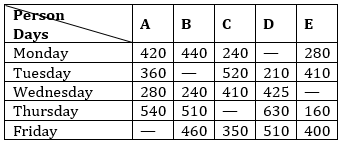Note: Some data are missing, calculate the missing data if required.

Q1. If total item sold by A and B including all five days is 2000 and 2200 respectively. Then item sold by B on Tuesday is what percent more/less than item sold by A on Friday ?
(a) 37.5%
(b) 23.5%
(c) 32.25%
(d) 42.5%
(e) 47%

Q2. If ratio of items sold by B and C together on Thursday to items sold by C and E together on same day is 2 : 1. Then find item sold by C on Thursday ?
(a) 150
(b) 210
(c) 190
(d) None of these
(e) 170

Q3. What is difference of item sold by A on Monday and Friday. If total item sold by A is 1800 ?
(a) 320
(b) None of these
(c) 225
(d) 210
(e) 220

Q4. If average of items sold by D on Monday and Tuesday is 245. Then items sold by D on Monday is what percent of item sold by E on Friday ?
(a) None of these
(b) 50%
(c) 60%
(d) 70%
(e) 75%

Q5. If items sold by C on Thursday is average of items sold by C on Wednesday and Friday then find total items sold by C ?
(a) 1750
(b) 1900
(c) 1700
(d) None of these
(e) 1850

Directions (6-10): Study the passage carefully and answer the questions based on it.
Marks obtained by X in Physics is 160 which is 40% of total marks obtained by him. And marks obtained by Z in chemistry is ¾th of marks obtained by X in Physics. Ratio of total marks obtained by X to Z is 4 : 3. Marks obtained by Y in Maths is equal to marks obtained by Z in Chemistry. And total marks obtained by Y in Physics and Chemistry together is half of total marks obtained by X.

Q6. If marks obtained by X in Maths is 50% more than marks obtained by him in Chemistry. Then find difference of marks obtained by X in Maths and marks obtained by Y in Maths ?
(a) 12
(b) 14
(c) 24
(d) 32
(e) 28

Q7. Marks obtained by Y in Physics and Chemistry together is what percent of total marks obtained by Z ?
(a) 66 ⅔%
(b) 50%
(c) None of these
(d) 33 ⅓%
(e) 75%

Q8. What is ratio of marks obtained by Z in Physics and Maths together to marks obtained by X in Chemistry and Physics together ?
(a) 2 : 5
(b) 1 : 2
(c) cannot be determined
(d) 4 : 3
(e) 2 : 3

Q9. If Max. marks in each subject is 200. Then find percent of marks obtained by Y ?
(a) 61 ⅓%
(b) 42 ⅔%
(c) 53 ⅓%
(d) None of these
(e) 47 ⅔%

Q10. If ratio of marks obtained by Z in Physics and Maths is 2 : 1, then find average marks obtained by X and Z in Physics ?
(a) None of these
(b) 150
(c) 160
(d) 120
(e) 140

Directions (11-15): Line chart given below shows cost price of six different types of articles while table shows profit percent earned on selling that article. Study the data carefully and answer the following questions.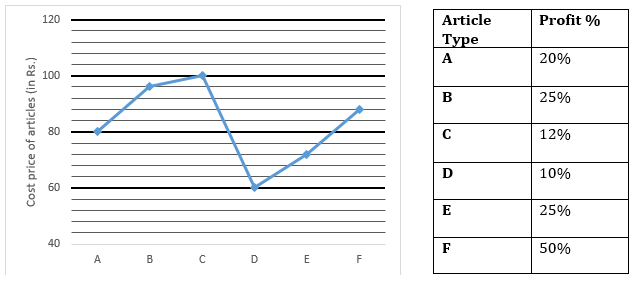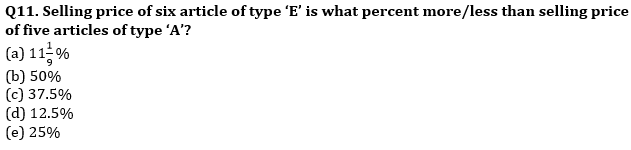Q12. Selling price of type ‘F’ is what percent of the selling price type ‘D’?
(a) 200%
(b) 100%
(c) 150%
(d) 50%
(e) 300%

Q13. Retailor sold five articles of type ‘B’ and two articles of type ‘A’. Find profit % earned by retailor?
(a) 19.75%
(b) 21.25%
(c) 23.75%
(d) 25.25%
(e) 27.75%

Q14. Find the average profit earned by retailor on selling one article of each type?
(a) 16
(b) 18
(c) 20
(d) 14
(e) 22

Q15. Find the ratio between total selling price of type ‘A’ and ‘B’ together to total selling price of type ‘D’ and ‘F’ together?
(a) 9 : 11
(b) 12 : 11
(c) 6 : 5
(d) 13 : 11
(e) 7 : 5

Solutions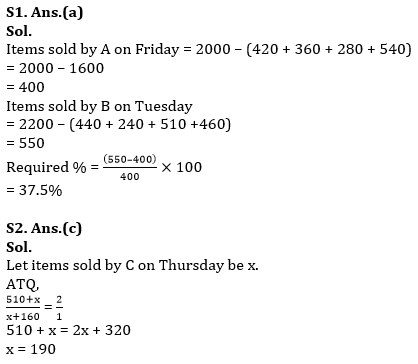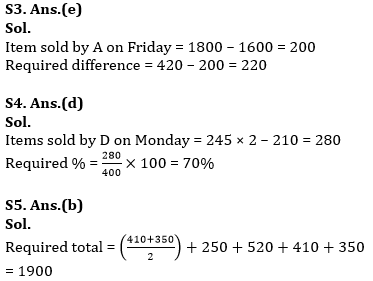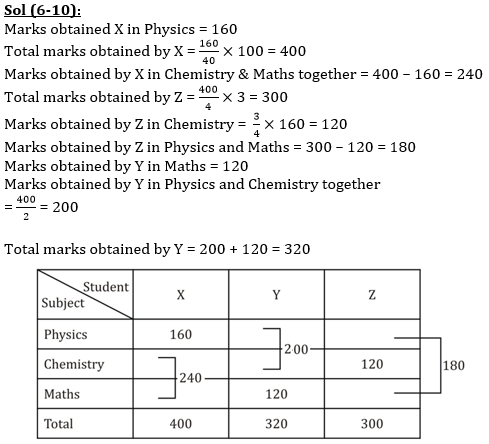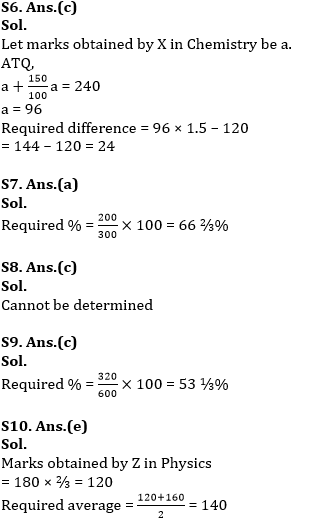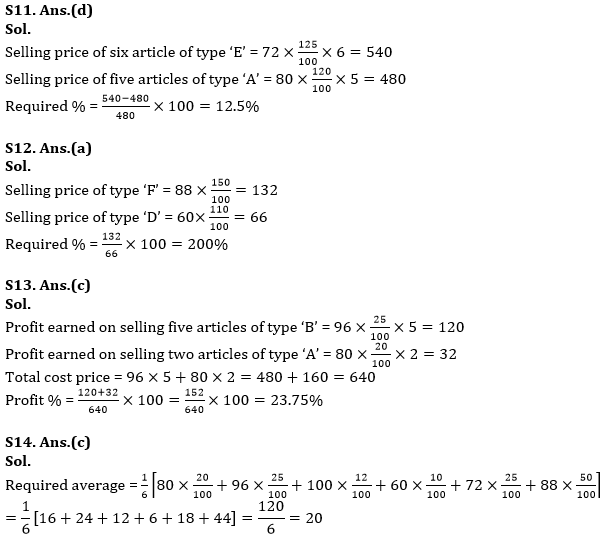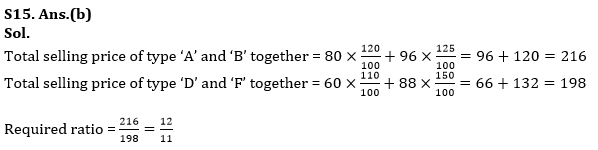#### Congratulations!Union Budget 2023-24: Free PDF